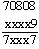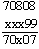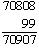Date: Sun, 14 Feb 1999 12:50:21 EST Subject: Small Numbers Find the smallest number that must be added to 70808 so that the digits would read the same backward or forward. Hi A number that reads the same forward and backward is called a palindrome.    You can certainly add 10000 to get 80808, but is there something you can add and keep the first digit a 7? If so the last digit of the sum must be a 7 also so the last digit of the number added must be a 9. Hence you know that the sum would look likewhere the digits marked x are yet to be determined.    If the sum is to be as small as possible can its second digit be 0? If so the fourth digit of the sum would have to be a 0 also and hence the sum would beThis sum can then be completed asCheers Penny Go to Math Central To return to the previous page use your browser's back button.